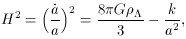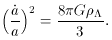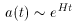In past years, inflation has become more of a scenario than model. A plethora of models have been suggested, all of which share the common feature that the universe goes through a brief period of rapid expansion. This rapid expansion is manifested in the evolution of the scale factor, a(t). In the case of inflation, a(t) ~ tn, where n > 1 and the universe expands faster than light. This does not violate relativity, since the spacetime is the thing expanding (i.e., no information is being transferred). Since n can take on any value greater than one, this is already an example of the flexibility of the theory.

In Section 2 it was shown by Equation (14) that if an equation of state p = -is achieved and one has a positive cosmological constant, then the universe will accelerate. Incorporating the cosmological constant,, into an energy density,, and assuming it is the dominate one can use (15) and (16) to obtain,(45)

One can choose to ignore the curvature term, since one anticipates a large increase in the scale factor. That is, the presence of the scale factor in the denominator of the -k/a2 term in the equation above will leave this term negligible. This is often referred to as the redshifting of the curvature, since the effect of the curvature can be ignored if the scale factor becomes large enough during a period of constant energy density. This is actually a glimpse of how the flatness problem will be resolved. So, ignoring the curvature term, we have(46)

This is a differential equation with the solution,(47)

where H = (8/3G)1/2 and sinceis a constant, so is H. This model is referred to as the DeSitter model (18) By introducing a negative pressure, the flatness problem is solved. The crux of this argument is thatis a constant (19) This comes from the fact thatis an intrinsic property of the spacetime manifold. As the manifold is stretched, this vacuum energy does not change. Another way this can be explained is by that the Einstein equations are arbitrary up to a constant term. The disadvantage of this explanation is that it does not manifest the connection between cosmology and particle theory (more on this later).

Sinceis taken to be the dominate form of energy, the other contributions to the density in the Friedmann equation (28) are also redshifted away, sinceM ~ a-3,R ~ a-4. This leads to the conclusion that no matter what the initial distribution ofT =M +R +, the vacuum energy will eventually dominate. Thus, the assumption ofdomination can actually be relaxed.

So given a constant vacuum term, the DeSitter scenario `drives' the universe to a flat geometry, thus approaching=c, wherec is the critical density, (i.e.,c = (3H2) / (8G). This evolution, if allowed to continue, will produce an empty universe with practically no radiation or matter. The fact that we live in a universe that is full of matter and radiation is why the original proposal, by DeSitter, was rejected and forgotten.

The revision of this idea was suggested by Guth in the early 1980's , . The crux to the modern inflationary scenario, in contrast to the DeSitter model, is to limit the amount of time that this rapid expansion (inflation) occurs. Guth explained the physical mechanism for such an inflationary period as corresponding to a phase transition in the early universe. By limiting the time of the quasi-exponential expansion, Guth was able to produce a universe more like our own. Unlike DeSitter's model, which was based on a pure solution to Einstein's equations, Guth's idea was based on ideas from particle physics. Guth was studying a class of grand unified theories (GUTs) and the predictions they make about particle production in the universe. This suggested how cosmology could be united with particle physics in a phenomenological manner, which has become one of the most appreciated beauties in modern physics today.

18 Not to be confused with the Einstein-DeSitter model. Back.
19 Actually,does not have to be a constant; in-fact, it can be a function of time. Such vacuum energies are referred to as Quintessence, or Dark energy, and are the subject of much research. Unfortunately, time will not permit a discussion ( , ). Back.